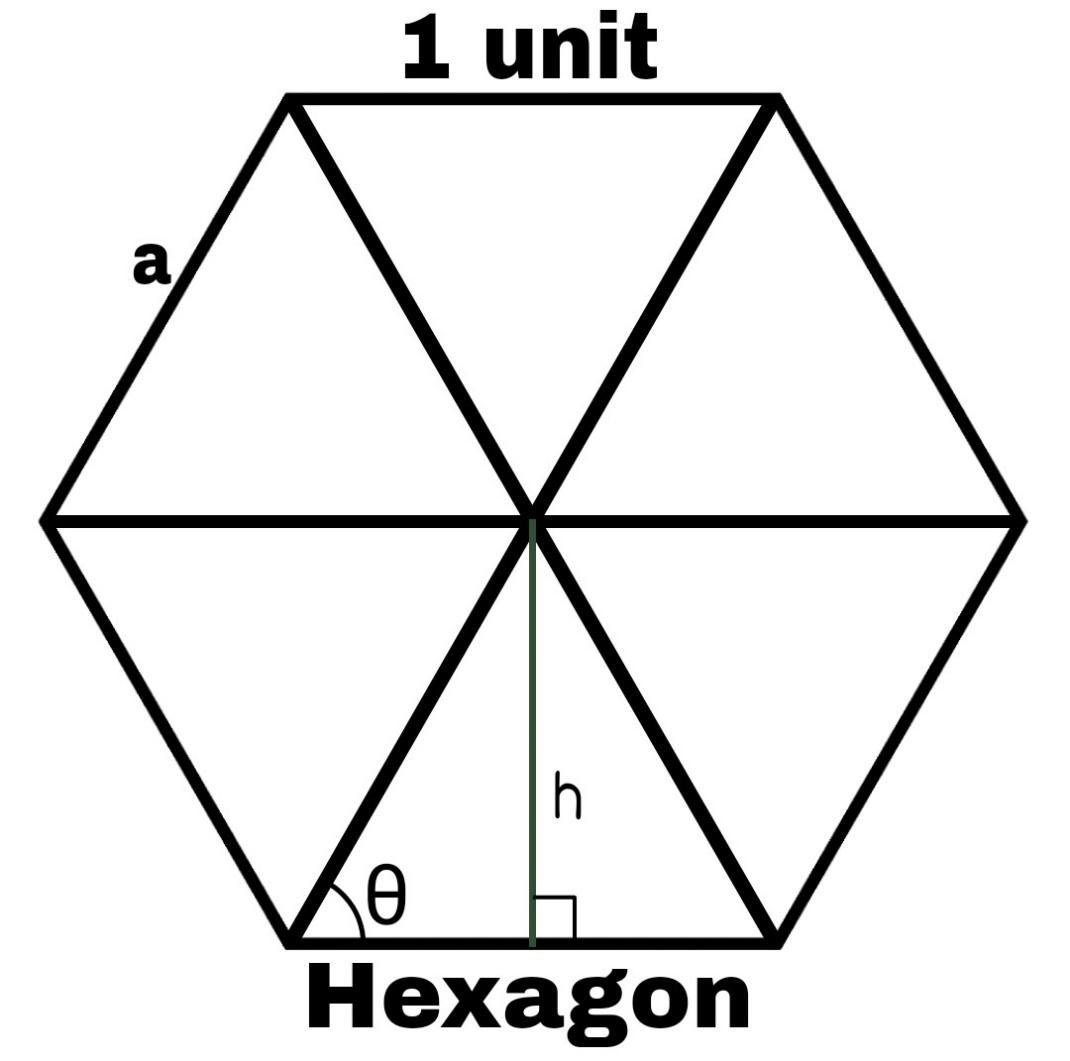# What is the area of a regular polygon of $n$ sides, each of which is $1$ unit?Verified
98.7k+ views
Hint: Try to simplify the problem by breaking the polygon into its simpler parts, note that every regular polygon is a combination of the same isosceles triangles. If we were able to find the area of one of the isosceles triangles, we can easily find the total area of the polygon just by multiplying the area of one triangle with the number of triangles.

In order to simplify and elaborate the problem we have taken a hexagon as an example
Now $a$ is the side of the hexagon whose length is $1$, the height of the perpendicular from the centre of the polygon to one of the side is $h$ which is the midpoint of the isosceles triangle. The equal angle of the isosceles triangle is $\theta$.Now we can see that as we divide the hexagon it splits into six isosceles triangles, and this result is same for all regular polygons. Every time we divide a regular polygon with $n$ sides it splits itself into $n$ isosceles triangles.
Now the total area of the hexagon is 6 times the area of the isosceles triangle.
Before calculating the area, we need to find its base and height. The base is length of the side of the polygon and for height we need to find the value of $\theta$ .
$\bullet$ We know that when a line from the centre of the polygon is joined with the vertex of the polygon it divides the angle of the polygon into two equal halves, so $\theta$ is half the angel of the polygon
$\bullet$ And the angle of the polygon $= \dfrac{{{{180}^ \circ } - \left( {\dfrac{{{{360}^ \circ }}}{n}} \right)}}{2}$
$\bullet$ So the angle $\theta = \dfrac{{{{180}^ \circ } - \left( {\dfrac{{{{360}^ \circ }}}{n}} \right)}}{2}$
$\bullet$ $\theta = {90^ \circ } - \left( {\dfrac{{{{180}^ \circ }}}{n}} \right)$
Now by applying simple trigonometry we need to find the height of the triangle:
$\bullet$ $\tan \theta = \dfrac{{perpendicular}}{{base}}$
$\bullet$ Now the perpendicular is $h$ and the base is $\dfrac{a}{2}$
$\bullet$ $\tan \theta = \dfrac{h}{{\left( {\dfrac{a}{2}} \right)}}$
$\bullet$ $h = \left( {\dfrac{a}{2}} \right) \times \tan \theta$
$\bullet$ Now by substituting the values of $\theta$ we get
$\bullet$ $h = \left( {\dfrac{a}{2}} \right) \times \tan \left( {{{90}^ \circ } - \left( {\dfrac{{{{180}^ \circ }}}{n}} \right)} \right)$
$\bullet$ Now the value of $\tan \left( {{{90}^ \circ } - \theta } \right) = \cot \theta$
$\bullet$ $h = \left( {\dfrac{a}{2}} \right) \times \cot \left( {\dfrac{{180 \circ }}{n}} \right)$
Since we have the height $h = \left( {\dfrac{a}{2}} \right) \times \cot \left( {\dfrac{{180 \circ }}{n}} \right)$and $base = a$ we can get the area of the total by substituting the values:
$\bullet$ Area $= n \times \dfrac{1}{2} \times \left( {base} \right) \times \left( {height} \right)$
$\bullet$ Area $= n \times \dfrac{1}{2} \times \left( a \right) \times \left( {\left( {\dfrac{a}{2}} \right) \times \cot \left( {\dfrac{{180 \circ }}{n}} \right)} \right)$
$\bullet$ Area $= \dfrac{n}{4}\left( {{a^2} \times \cot \left( {\dfrac{{{{180}^ \circ }}}{n}} \right)} \right)$
$\bullet$ Since the value of $a = 1$ unit
$\bullet$ Area $= \dfrac{{n\cot \left( {\dfrac{{{{180}^ \circ }}}{n}} \right)}}{4}\;\;{\left( {unit} \right)^2}$
Thus the area of a regular polygon of $n$ sides, each of which is $1$ unit is $\dfrac{{n\cot \left( {\dfrac{{{{180}^ \circ }}}{n}} \right)}}{4}\;\;{\left( {unit} \right)^2}$

Note: In order to simplify the problems especially related to geometry such as area we can divide it into simple shapes like triangle or square to make it easy, this method is widely used to simplify complex geometrical figures into smaller units which are very easy to deal with. And the above formula which we derived can be used for polygons with any number of sides, but it must be a regular one.